# Solving Matrices and Absolute Value: Tutoring Solution Chapter Exam

Exam Instructions:

Choose your answers to the questions and click 'Next' to see the next set of questions. You can skip questions if you would like and come back to them later with the yellow "Go To First Skipped Question" button. When you have completed the practice exam, a green submit button will appear. Click it to see your results. Good luck!

Answered 0 of 30

### Page 2

#### Question 6 6. What size is the following matrix?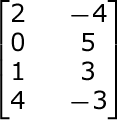#### Question 10 10. Which system of equations is represented by the following matrix? (Think of the vertical line as the equal sign.)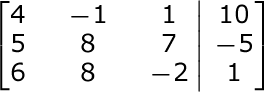### Page 3

#### Question 11 11. Which equation matches the graph below?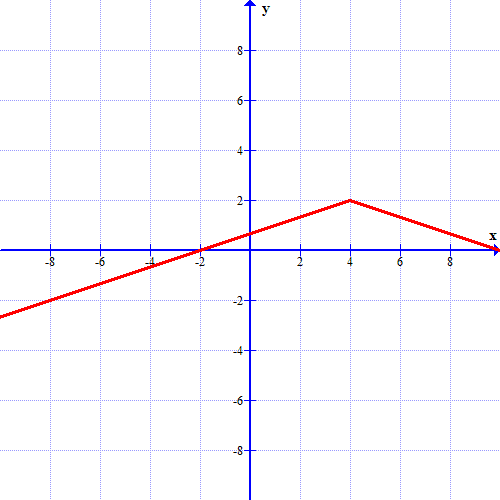#### Question 12 12. Which graph matches the equation below?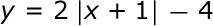#### Question 13 13. Find the determinant of the following matrix.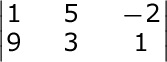### Page 5

#### Question 21 21. Which equation matches the graph below?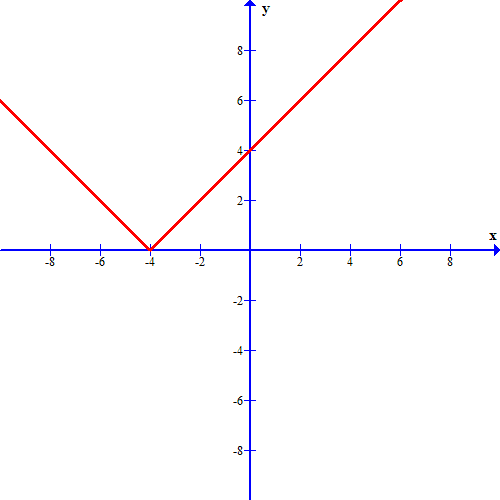#### Question 22 22. Which graph matches the equation below?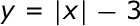### Page 6

#### Solving Matrices and Absolute Value: Tutoring Solution Chapter Exam Instructions

Choose your answers to the questions and click 'Next' to see the next set of questions. You can skip questions if you would like and come back to them later with the yellow "Go To First Skipped Question" button. When you have completed the practice exam, a green submit button will appear. Click it to see your results. Good luck!

Support